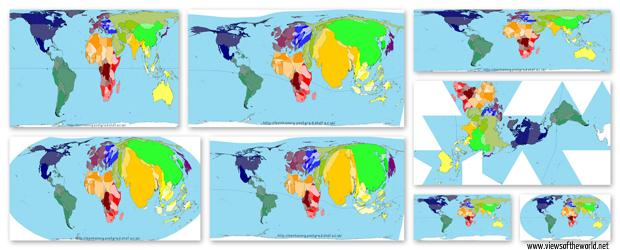(如需转载，请在显著位置注明个人微信公众号stdrei)

### EPSG Code

The CRSs available in QGIS are based on those defined by the European Petroleum Search Group (EPSG) and the Institut Geographique National de France (IGNF) and are largely abstracted from the spatial reference tables used in GDAL. EPSG identifiers are present in the database and can be used to specify a CRS in QGIS.

### pyproj小试牛刀

pyproj是PROJ4的python接口封装，直接看一个官网的例子吧。直接利用epsg code来定义投影参数。

``````from pyproj import Proj,transform

# The Proj class can convert from geographic (longitude,latitude)
# to native map projection (x,y) coordinates and vice versa,
# or from one map projection coordinate system directly to another.

p1 = Proj(init='epsg:26915')
p2 = Proj(init='epsg:26715')
x1, y1 = p1(-92.199881,38.56694)
x2, y2 = transform(p1,p2,x1,y1)
print '%9.3f %11.3f' % (x1,y1)
print '%9.3f %11.3f' % (x2,y2)
``````

``````569704.566 4269024.671
569722.342 4268814.027
``````

### 基于geopandas的矢量地图投影

``````import shapely, geopandas, fiona
import seaborn as sns
from fiona.crs import from_epsg,from_string

# Data
shp = 'E:\NationalGISdata\Province.shp'

shp_df = geopandas.GeoDataFrame.from_file(shp)
# #IndexError报错的话，用arcgis将shapefile文件重新导出一遍试试
``````
``````# 根据当前的兰伯特投影绘制
shp_df.plot(column="GDP_1994",colormap='Set1')
``````
``````# 转换到经纬度坐标
shp_df_wgs84 = shp_df.to_crs(from_epsg(4326))
shp_df_wgs84.plot(column="GDP_1994",colormap='Set1')
``````
``````# 国家2000坐标系
# EPSG:4508  CGCS2000 / Gauss-Kruger CM 111E
shp_df_4508 = shp_df.to_crs(from_epsg(4508))
shp_df_4508.plot(column="GDP_1994",colormap='Set1')
``````
``````# 除了直接用ESPG code，也可以自己定义投影参数

ESRI_54024 = """
+proj=bonne +lon_0=0 +lat_1=60 +x_0=0 +y_0=0 +ellps=WGS84 +datum=WGS84 +units=m +no_defs

shp_df_3408 = shp_df.to_crs(from_string(ESRI_54024))
shp_df_3408.plot(column="GDP_1994",colormap='Set1')
``````